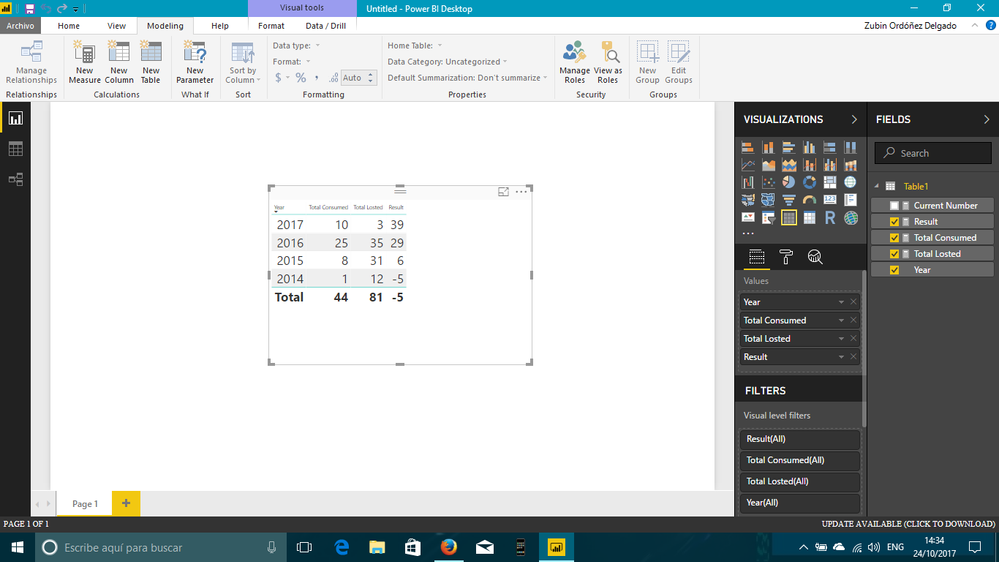cancel
Showing results for
Did you mean:Frequent Visitor

## Calculations with previous row

Hi guys,

I've the following dataset that shows me the numbe of consumed and losted tickets in last 4 years. And I know that I have 32 tickets consumed for the next year.

 Tickets_Consumed Year Tickets_Losted Year 10 2017 3 2017 25 2016 35 2016 8 2015 31 2015 1 2014 12 2014

And I'm trying to calculate the following formula in PowerBI:

 Tickets_Consumed Year Tickets_Losted Year Result 10 2017 3 2017 39 (32+10-3) 25 2016 35 2016 29(39+25-35) 8 2015 31 2015 6(29+8-31) 1 2014 12 2014 -5(6+1-12)

How do I get the result:

Current_Number of Tickets Consumed = 32
Number of Tickets Consumed;

Number of Tickets Losted;

How can I do this using PowerBI? I can share the excel to see what I'm calculating

Thanks

1 ACCEPTED SOLUTIONSolution Specialist

Try this measures...

`Total Consumed = SUM(Table1[Tickets Consumed]) `
`Total Losted = SUM(Table1[Tickets Losted]) `
`Current Number = 32 `
```Result = var actualyear = FIRSTNONBLANK(Table1[Year];1) return

CALCULATE([Current Number] + [Total Consumed] - [Total Losted];ALL(Table1);Table1[Year]>=actualyear) ```

and this is the final result...I hope this helps

Regards

BILASolution

5 REPLIES 5Solution Specialist

Try this measures...

`Total Consumed = SUM(Table1[Tickets Consumed]) `
`Total Losted = SUM(Table1[Tickets Losted]) `
`Current Number = 32 `
```Result = var actualyear = FIRSTNONBLANK(Table1[Year];1) return

CALCULATE([Current Number] + [Total Consumed] - [Total Losted];ALL(Table1);Table1[Year]>=actualyear) ```

and this is the final result...I hope this helps

Regards

BILASolutionHelper II

@BILASolution

Your solution is pretty slick. It looks simple but it's surprisingly little hard to undersatnd fully. Do you mind being so kind to explain how your code works:

```Result =
VAR actualyear = FIRSTNONBLANK ( Table1[Year]; 1 )
RETURN
CALCULATE (
[Current Number] + [Total Consumed] - [Total Losted];
ALL ( Table1 );
Table1[Year] >= actualyear
)```

ThanksSolution Specialist

The next picture is a summary of the logic that I used...I hope you could understandRegards

BILASolutionCommunity Champion

This calculated column shall get you desired result hopefully.

Modify Table Name

```Result=
32
+ CALCULATE (
SUM ( Table1[Tickets_Consumed] ),
FILTER ( Table1, Table1[Year] >= EARLIER ( Table1[Year] ) )
)
- CALCULATE (
SUM ( Table1[Tickets_Losted] ),
FILTER ( Table1, Table1[Year] >= EARLIER ( Table1[Year] ) )
)```

Regards
ZubairCommunity ChampionRegards
Zubair